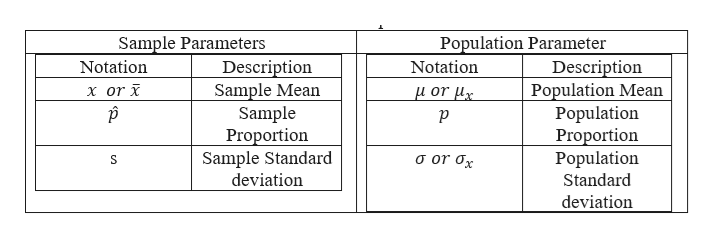# List two unbiased estimators and their corresponding parameters. (Select all that apply.)p is an unbiased estimator for p̂σ is an unbiased estimator for σxp̂ is an unbiased estimator for px is an unbiased estimator for μσx is an unbiased estimator for σμ is an unbiased estimator for x

Question

List two unbiased estimators and their corresponding parameters. (Select all that apply.)

p is an unbiased estimator for p̂σ is an unbiased estimator for σxp̂ is an unbiased estimator for px is an unbiased estimator for μσx is an unbiased estimator for σμ is an unbiased estimator for x
check_circleExpert Solution
Step 1

We need to list two unbiased estimators and their corresponding parameters. (Select all that apply.)

μ is an unbiased estimator for x

p̂ is an unbiased estimator for p

p is an unbiased estimator for p̂

x is an unbiased estimator for μ

σx is an unbiased estimator for σ

σ is an unbiased estimator for σx

Step 2

Please find the table notations an...help_outlineImage TranscriptionclosePopulation Parameter Description Population Mean Population Proportion Population Standard Sample Parameters Notation Description Sample Mean Sample Proportion Sample Standard deviation Notation x or μor μ S deviation fullscreen

### Want to see the full answer?

See Solution

#### Want to see this answer and more?

Solutions are written by subject experts who are available 24/7. Questions are typically answered within 1 hour*

See Solution
*Response times may vary by subject and question
Tagged in

### Probability